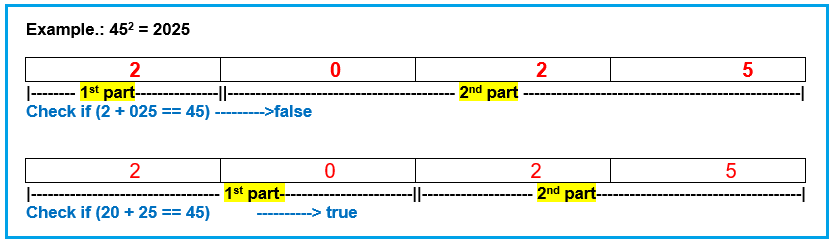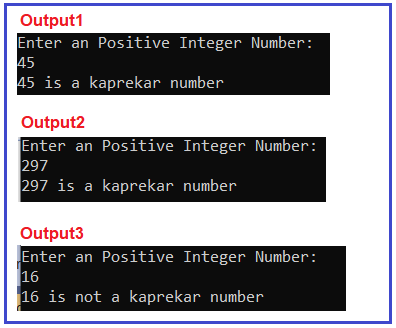# Kaprekar Number in C#

## Kaprekar Number in C# with Examples

In this article, I am going to discuss Kaprekar Number Program in C# with Examples. Please read our previous article where we discussed the Strong Number Program in C#. Here, in this article, first, we will learn what is a Kaprekar Number and then we will see how to implement the Kaprekar Number Program in C#. And finally, we will see how to print all the Kaprekar numbers between a range of numbers like between 1 to 100 or 100 to 1000, etc.

##### What is a Kaprekar Number?

A number is said to be a Kaprekar number if its square divided into two parts and the sum of the parts will be equal to the original number.
Example: 45 is a Kaprekar number.
Explanation: 452 = 2025 and 20+ 25 = 45 (which is equal to the original number).

Example: 297 is a Kaprekar number.
Explanation: 2972 = 88209 = 88+ 209 = 297 (which is equal to the original number).

Example: 16 is not a Kaprekar number.
Explanation: 162 = 256 and Neither 25 + 6 nor 2 + 56 is equal to 16

##### Points to Remember while working with Kaprekar Number:
1. Condition1: The square of number should be divided into only two parts (not more than 2 or not less than 2).
2. Condition2: The two parts are not necessary to equal in count (the digits of two parts may be different or maybe equal we need to check all combinations to verify).
3. Condition3: None of the parts should be equal to 0. It may contain 0 as a digit but should be greater than 0 as a whole part. For example, 19+70 is a valid two-part but 11+0 is not because the 2nd part has only 0 (it should be greater than 0).
##### How to implement Kaprekar Number Program in C#?

We are going to use the very basic method to implement this program but before that, you should have some basic knowledge of the following operators.

1. Modulus (%) Operator.
2. Divide (/) Operator.
3. Math.Pow(base,power).

In case if you are unfamiliar or not confident about these let’s have a look at our previous questions where we have discussed these in great detail like Duck Number, Disarium Number, Buzz Number, and Strong Number, etc.

###### Solution

Step1: Take a number from the user.
Step2: Find the square of number by just multiplying it with the number itself.
Step3: Count the number of digits in the square. For this, we are going to use the divide operator. We will divide the number by 10 each time until the number becomes equal to 0 and count them as the number of digits.
Example.: 432
432/10 = 43 –> count =1
43/10 = 4 –> count =2
4/10 = 0 –> count =3
So, the number 432 has 3 digits.

###### Step4: Find the two parts of the squared number

Find the two parts of the squared number. As the two parts should be of different digits in count (it’s not necessary that the two parts should be equal to the same digits count) we need to loop through the squared number one by one.

First, take the first digit of the number as a first part and the rest will be the second part. Take the first 2 digits of the number as a first part and again rest digits will be the second part. The list will go on depends on the number of digits present in the number.This list will go on if the condition does not become true or the number of digits in part1 becomes equal to the number of digits itself.

Step5: In the meanwhile, in Step4 we also took care of one of the main conditions of kaprekar number which is any of the parts should not equal 0. If yes then skip the iteration using the continue statement.
Step6: Calculate the sum of both parts.
Step7: Compare sum and number, if both are equal then the entered number is Kaprekar number otherwise, it is not.

##### Example: Kaprekar Number Program in C#.

The following C# Program will allow the user to input a number and then it checks whether that number is Kaprekar Number or not.

```using System;
public class KaprekarNumber
{
public static void Main()
{
Console.WriteLine("Enter an Positive Integer Number:: ");

// check the number is kaprekar number or not
if (IsKaprekar(number))
Console.WriteLine(number + " is a kaprekar number");
else
Console.WriteLine(number + " is not a kaprekar number");

// wait for user confirmation to exit
}

public static bool IsKaprekar(int number)
{
// declare variables
int square = 0;
int temp = 0;
int countDigits = 0;
int firstPart = 0;
int secondPart = 0;
int sum = 0;

// calculate square value of the number, Step2
square = number * number;

// count number of digits in the square, step 3
temp = square;
while (temp != 0)
{
countDigits++;
temp /= 10;
}

//Divide square into two parts and
//Check it's sum is equal to the number? Step4
for (int i = countDigits - 1; i > 0; i--)
{
// find first part
// first time it will divide the square by 10 then 100 then 100 and so on.
firstPart = square / (int)Math.Pow(10, i);

//find second part
secondPart = square % (int)Math.Pow(10, i);

//heck have any part only 0, Step 5
if (firstPart == 0 || secondPart == 0)
continue;

// find sum value, step 6
sum = firstPart + secondPart;

// compare sum and number, step 7
if (sum == number)
{
return true;
}
}
return false;
}
}
```
###### Output:##### C# Program to Print all Kaprekar Numbers Between 1 and N

The following C# Program will allow the user to input a number. Then it will print all the Kaprekar numbers between 1 and that input number.

```using System;
public class KaprekarNumber
{
public static void Main()
{
Console.WriteLine("Enter an Positive Integer Number: ");

Console.WriteLine("Kaprekar Numbers Between 1 and " + number + " : ");
for (int i = 1; i <= number; i++)
{
// check the number is kaprekar number or not
if (IsKaprekar(i))
{
Console.Write(i + " ");
}
}

// wait for user confirmation to exit
}

public static bool IsKaprekar(int number)
{
// declare variables
int square = 0;
int temp = 0;
int countDigits = 0;
int firstPart = 0;
int secondPart = 0;
int sum = 0;

// calculate square value of the number, Step2
square = number * number;

// count number of digits in the square, step 3
temp = square;
while (temp != 0)
{
countDigits++;
temp /= 10;
}

//Divide square into two parts and
//Check it's sum is equal to the number? Step4
for (int i = countDigits - 1; i > 0; i--)
{
// find first part
// first time it will divide the square by 10 then 100 then 100 and so on.
firstPart = square / (int)Math.Pow(10, i);

//find second part
secondPart = square % (int)Math.Pow(10, i);

//heck have any part only 0, Step 5
if (firstPart == 0 || secondPart == 0)
continue;

// find sum value, step 6
sum = firstPart + secondPart;

// compare sum and number, step 7
if (sum == number)
{
return true;
}
}
return false;
}
}
```
###### Output:In the next article, I am going to discuss How to Implement Krishnamurthy Number Program in C#. Here, in this article, I try to explain How to Implement the Kaprekar Number Program in C# with Examples and I hope you enjoy this Kaprekar Number Program in the C# article.